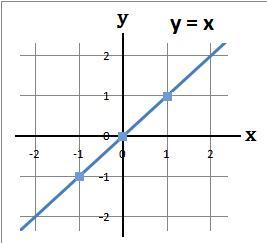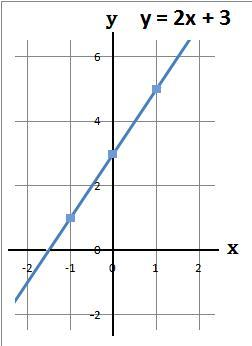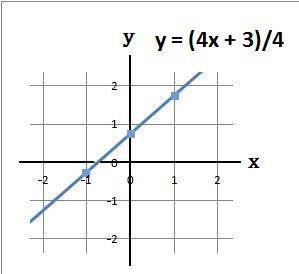### Straight Line Graphs

Straight line graphs are usually of the form:

 (1) y = ax + c c is where the straight line graph crosses the y axis (2) ax + by = c c/b is where the straight line graph crosses the y axis. For this type of equation begin by making y the subject. This has already been done in: Solving Equations − Add and Subtract and Solving Equations − Multiply and Divide

#### Example 1.   Plot the graph y = x

(a) Using a table work out the values of y

 x −1 0 1 y = x −1 0 1

(b) The coordinates of the above are (−1, −1) (0, 0) and (1, 1)

(c) Plot the above coordinates and draw a line through these points#### Example 2.   Plot the graph y = x + 2

(a) Using a table work out the values of y

 x −1 0 1 y = x + 2 1 2 3

(b) The coordinates of the above are (−1, 1) (0, 2) and (1, 3)

(c) Plot the above coordinates and draw a line through these points#### Example 3.   Plot the graph y = 2x + 3

(a) Using a table work out the values of y

 x −1 0 1 2x −2 0 2 +3 +3 +3 +3 y = 2x + 3 1 3 5

(b) The coordinates of the above are (−1, 1) (0, 3) and (1, 5)

(c) Plot the above coordinates and draw a line through these points#### Example 4.   Plot the graph 4y − 4x = 3

(a) Make y the subject

(b) Using a table work out the values of y
y = (4x + 3)/4

 x −1 0 1 4x −4 0 4 +3 +3 +3 +3 4x + 3 −1 3 7 ÷4 ÷4 ÷4 ÷4 y −0.25 0.75 1.75

(c) The coordinates of the above are (−1, −0.25) (0, 0.75) and (1, 1.75)

(d) Plot the above coordinates and draw a line through these pointsto: